# Concise Selina Solutions for Class 9 Maths Chapter 13- Pythagoras Theorem

Selina Solutions are considered to be very useful when you are preparing for the ICSE Class 9 Maths exams. Here, we bring to you detailed solutions to the exercises of Selina Solutions for Class 9 Maths Chapter 13- Pythagoras Theorem. These answers have been devised by the subject matter experts as per the syllabus prescribed by the CISCE for the ICSE.

Here we have provided the complete solutions of Class 9 Maths Chapter 13- Pythagoras Theorem in PDF format. Students can avail these Selina solutions and download it for free to practice them offline as well.

### Download PDF of Selina Solutions for Class 9 Maths Chapter 13:-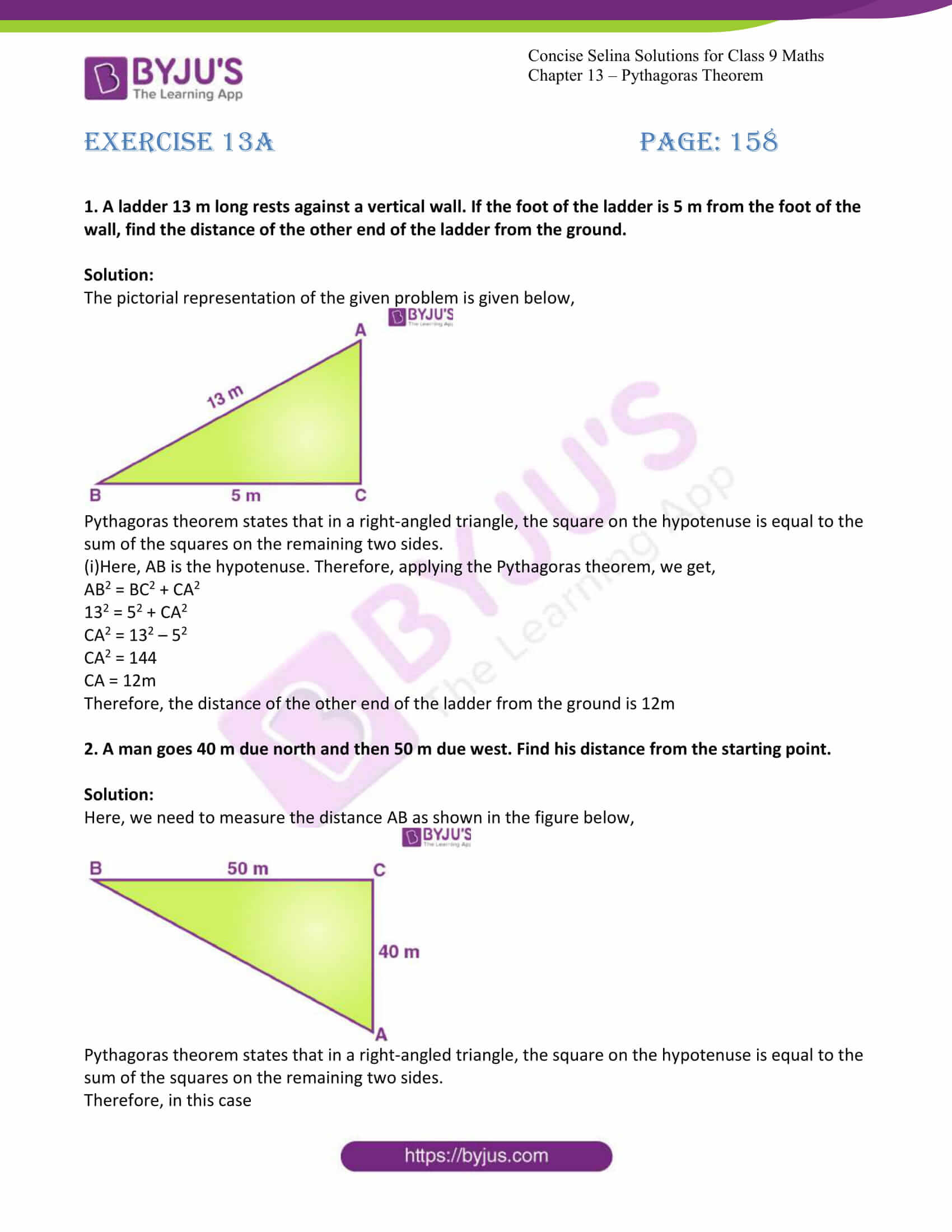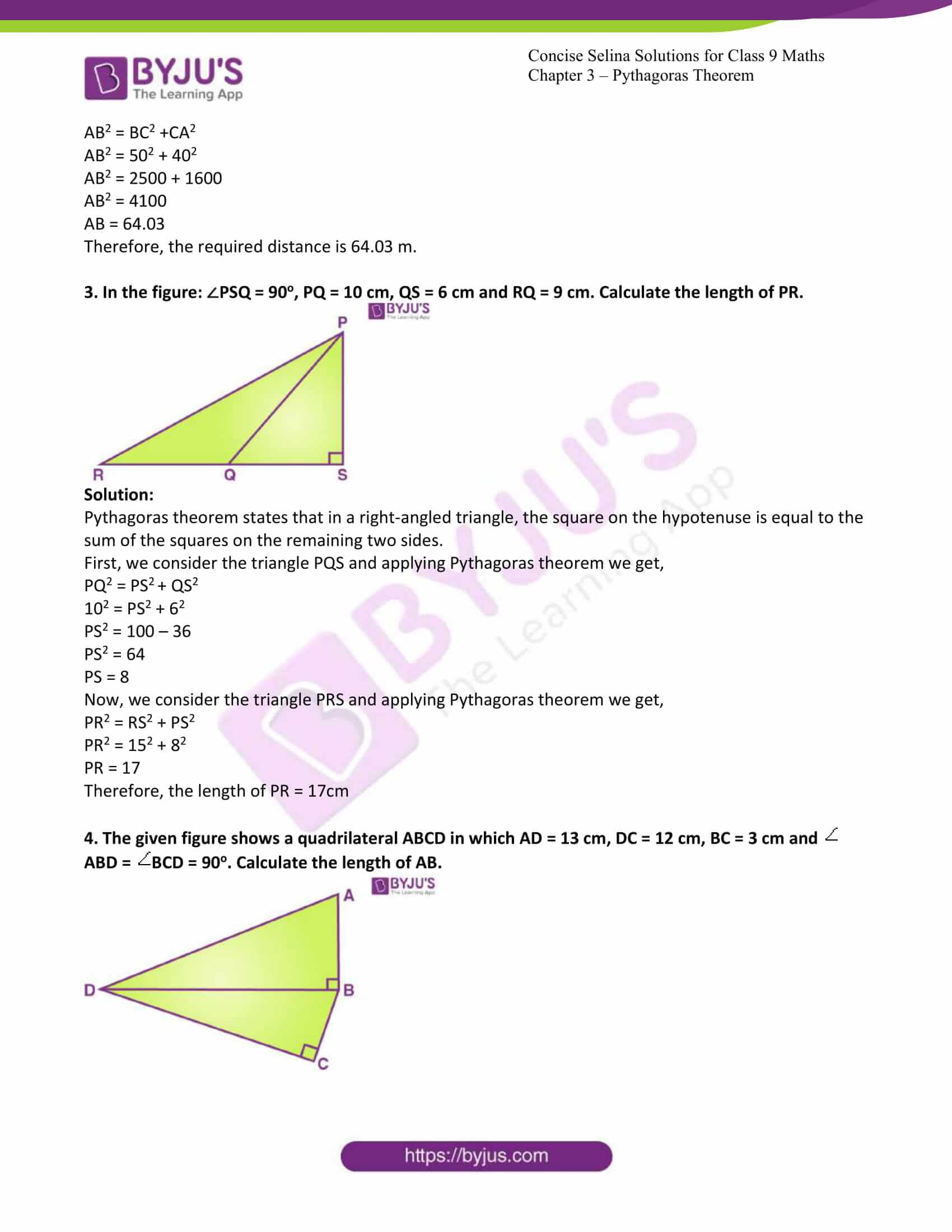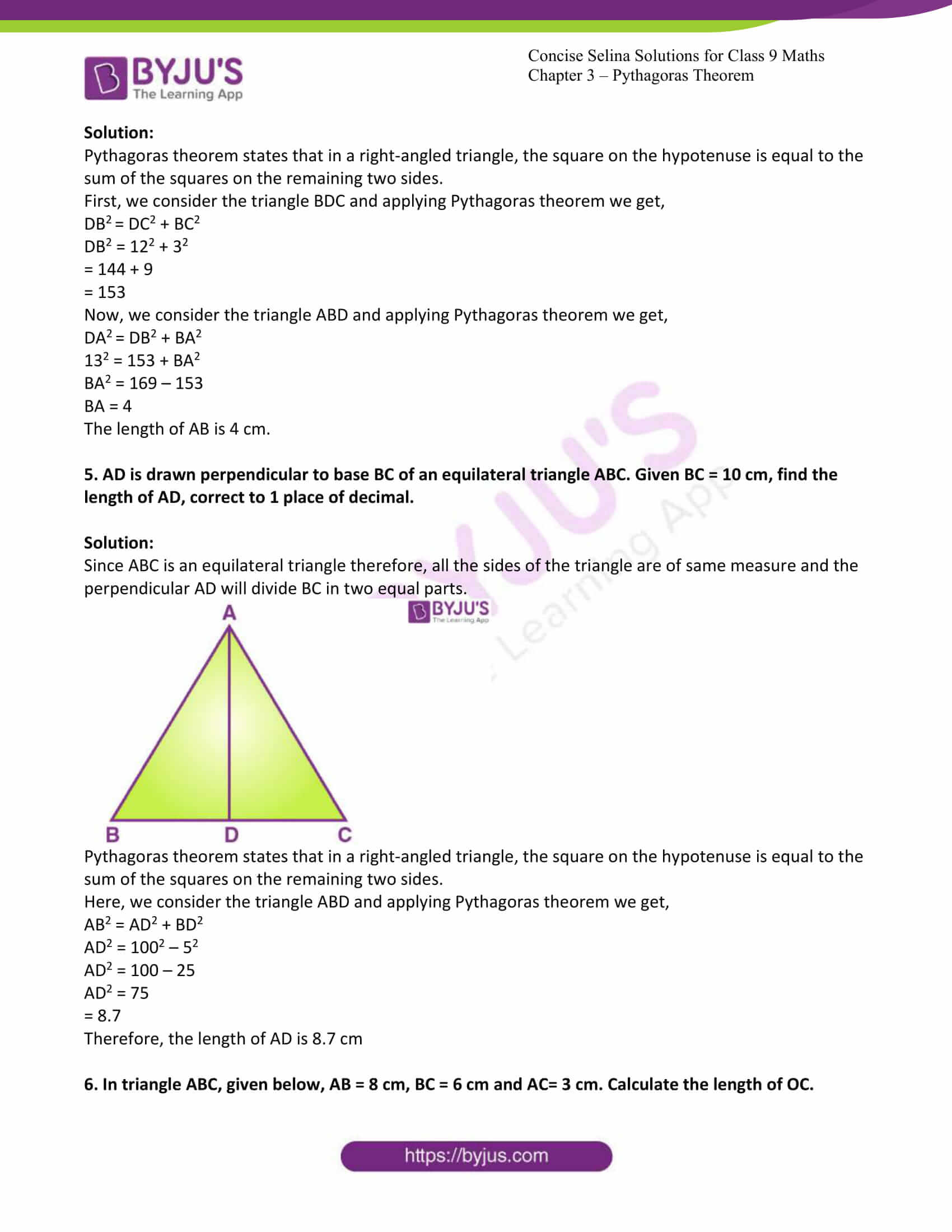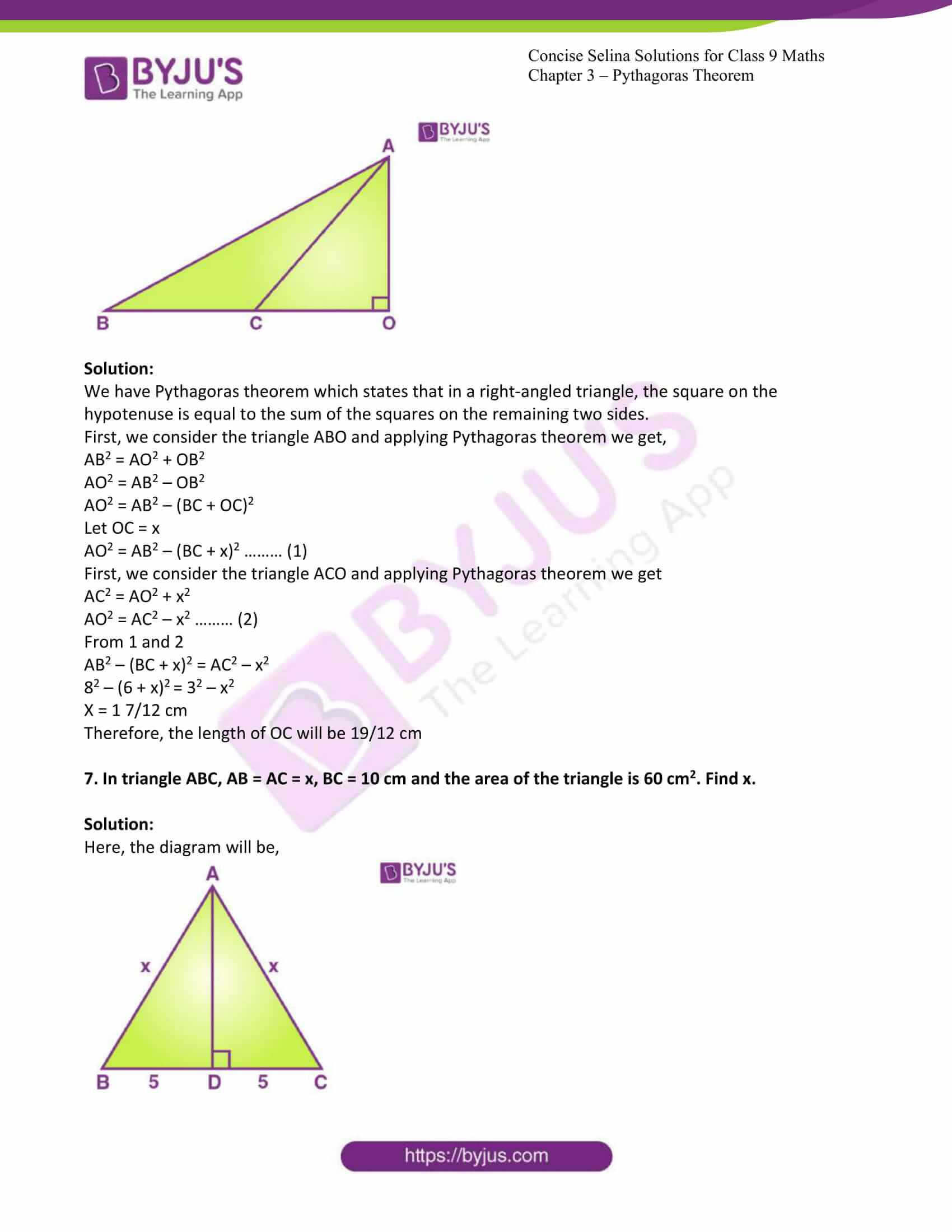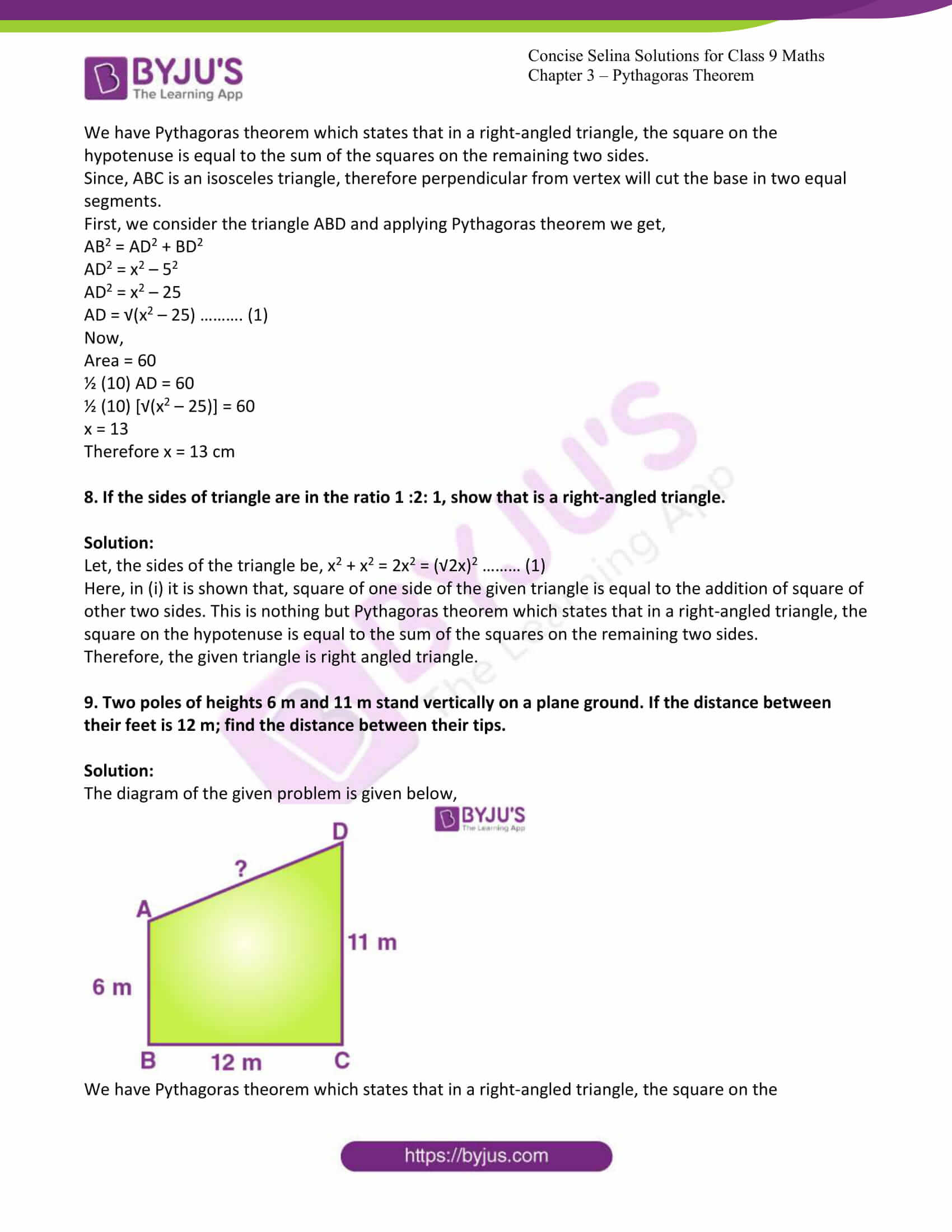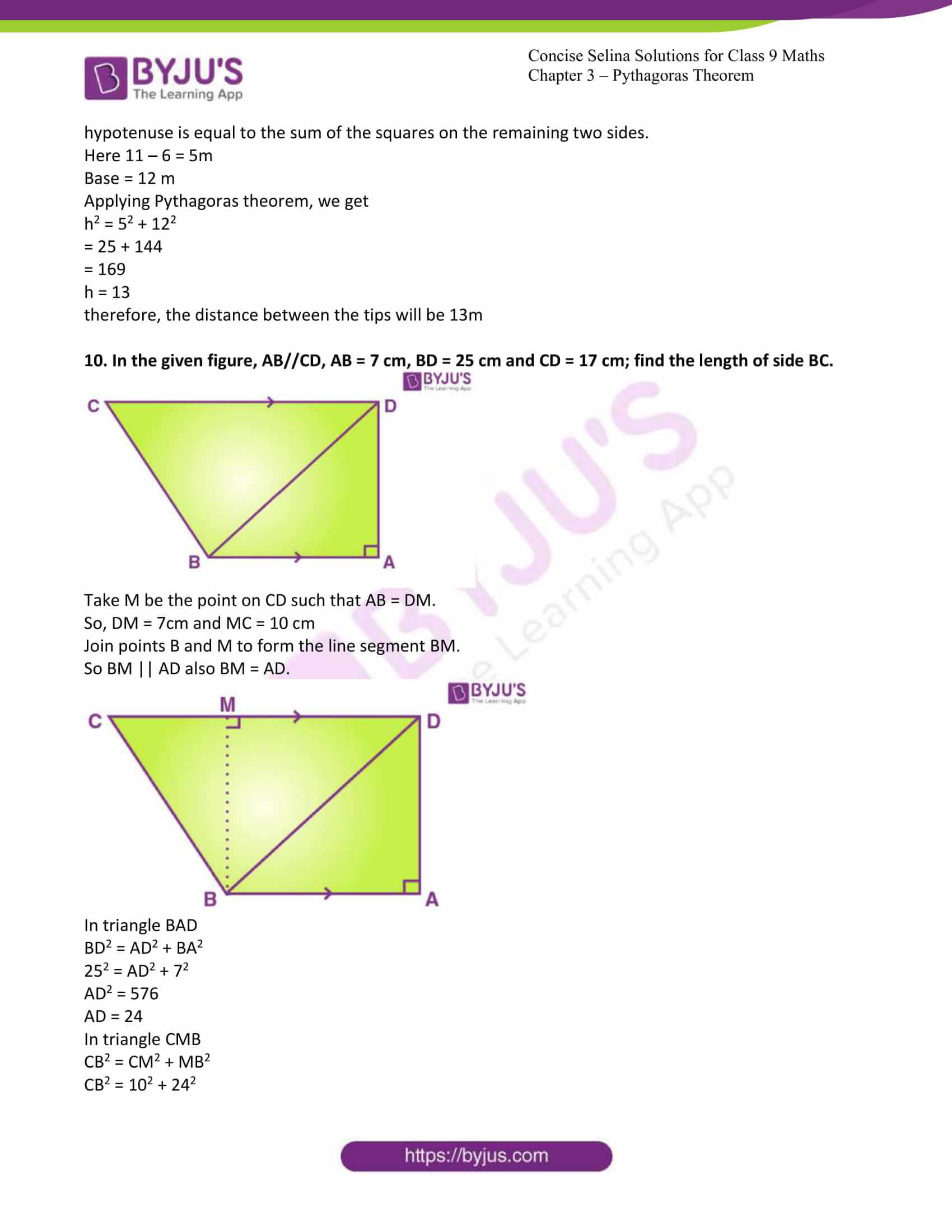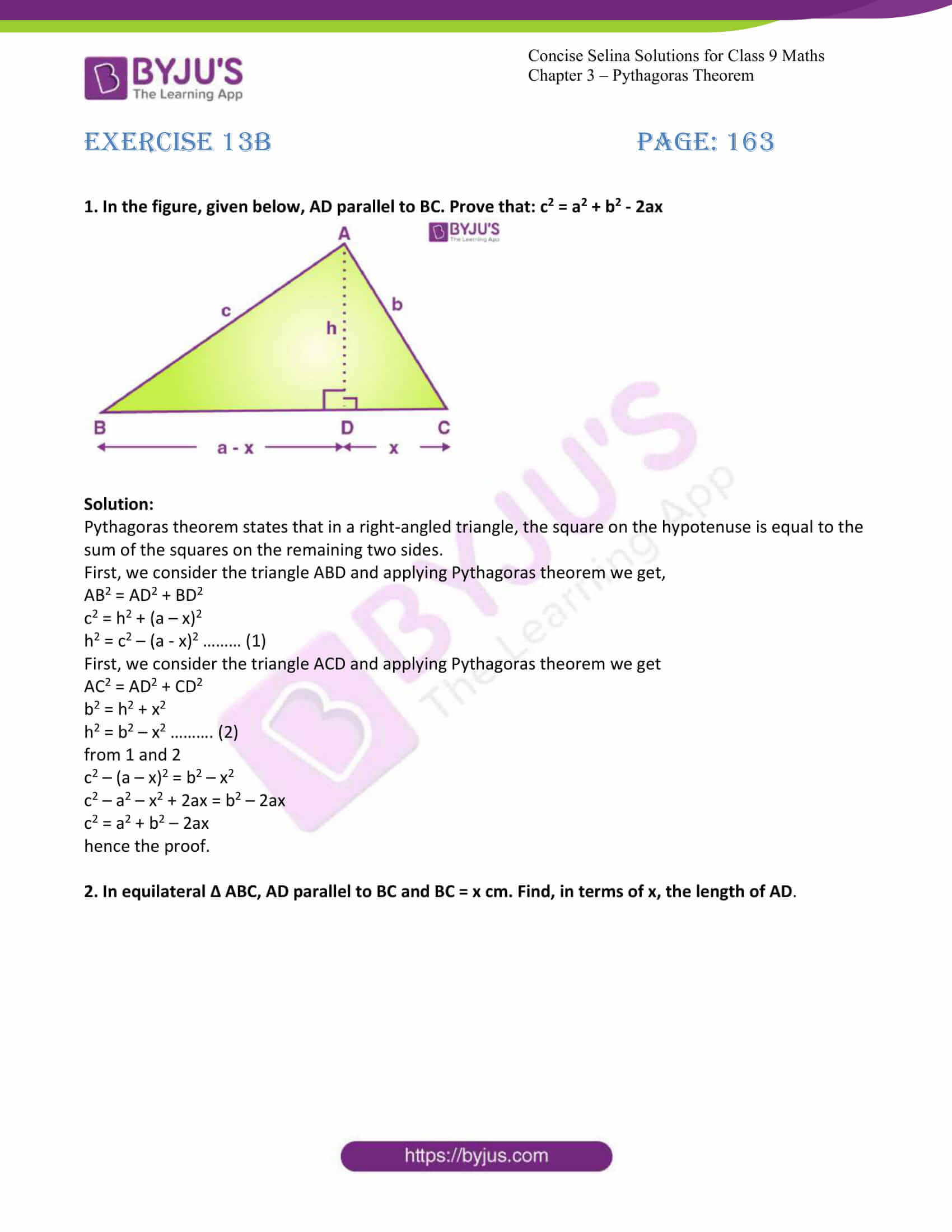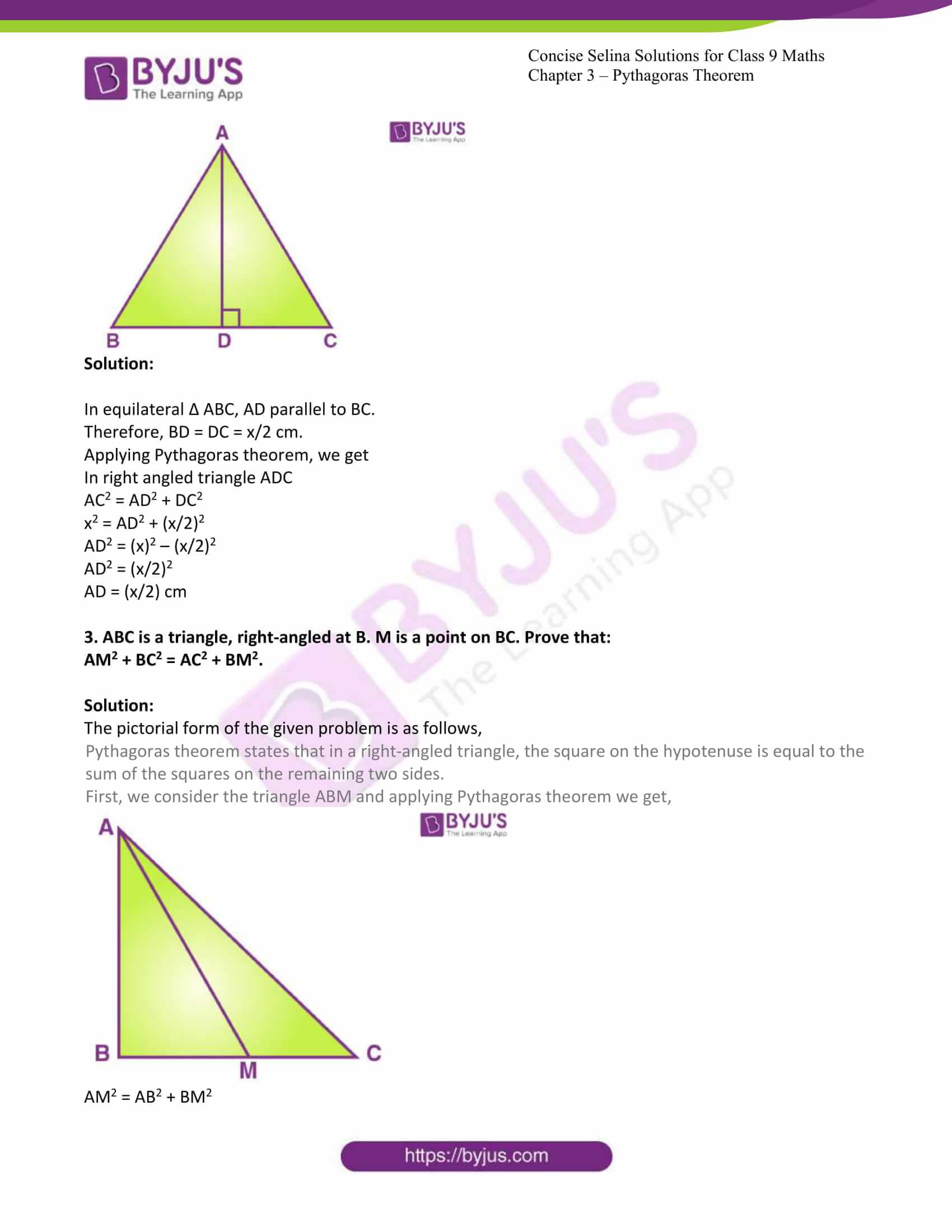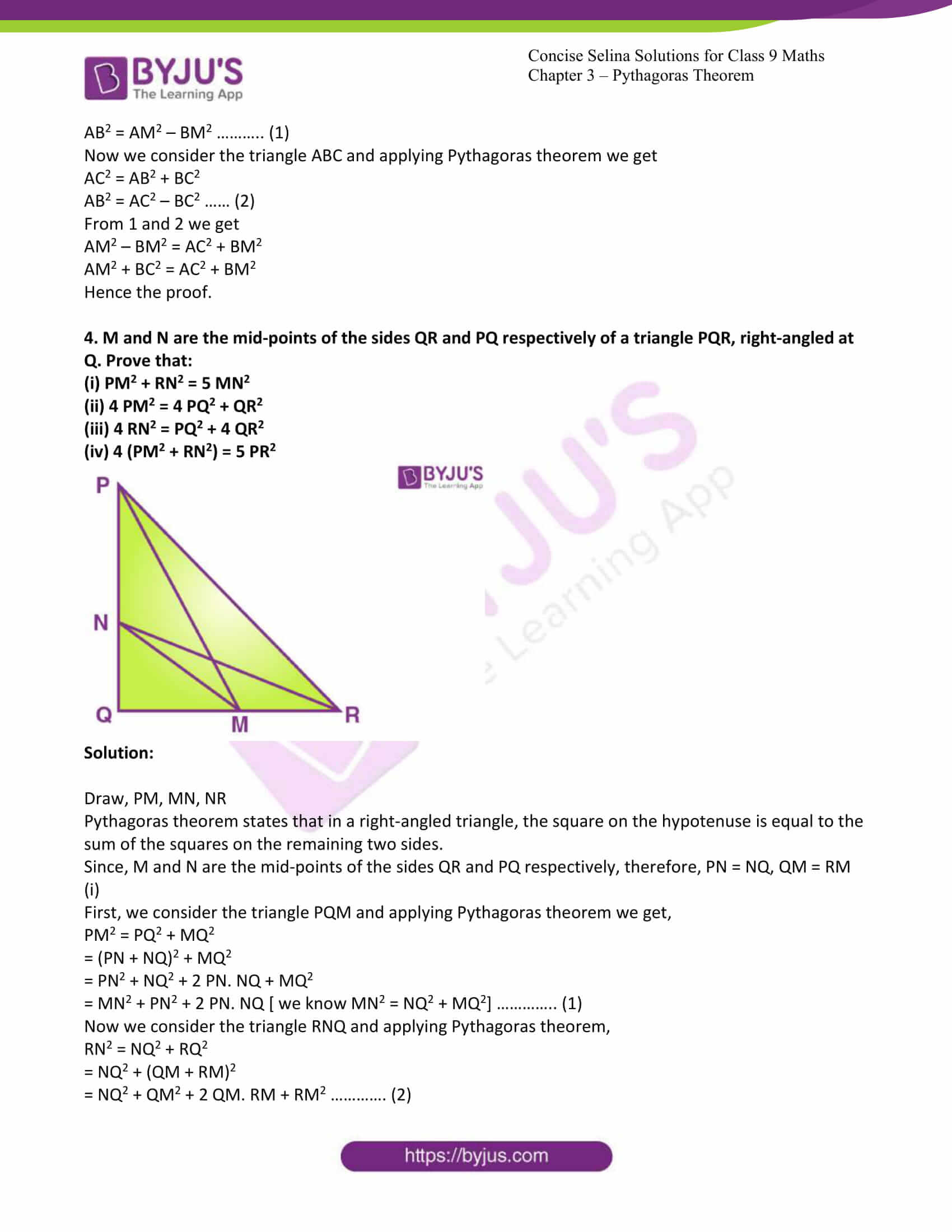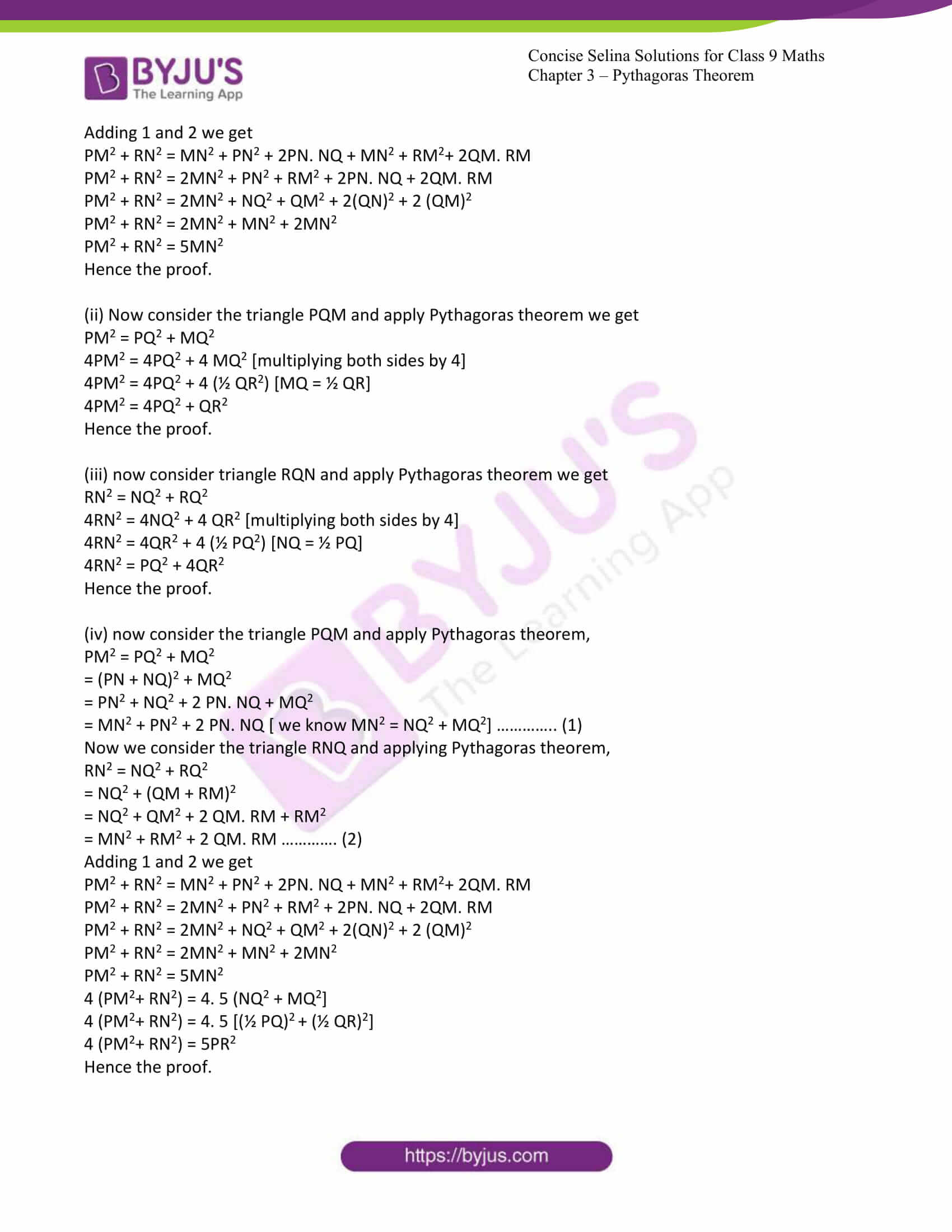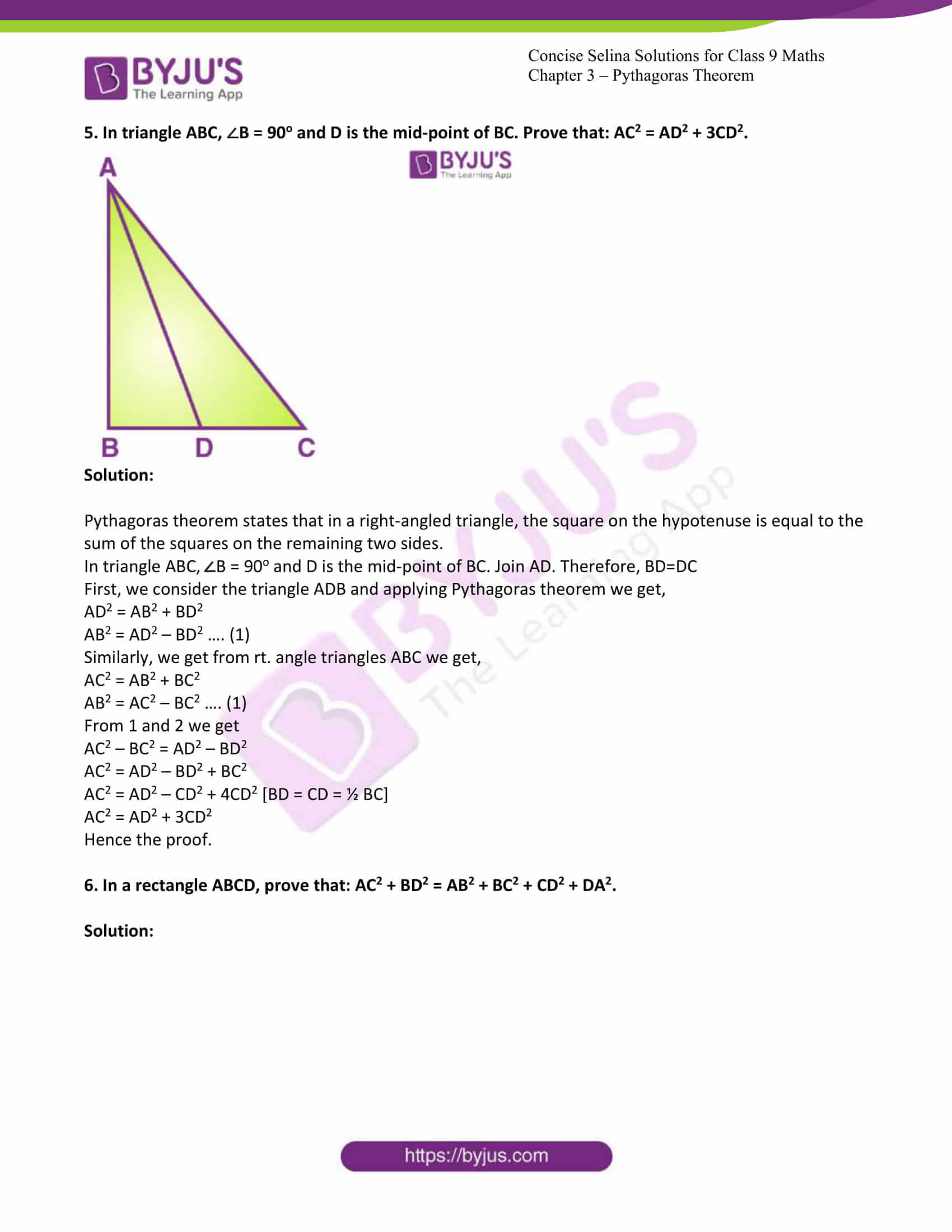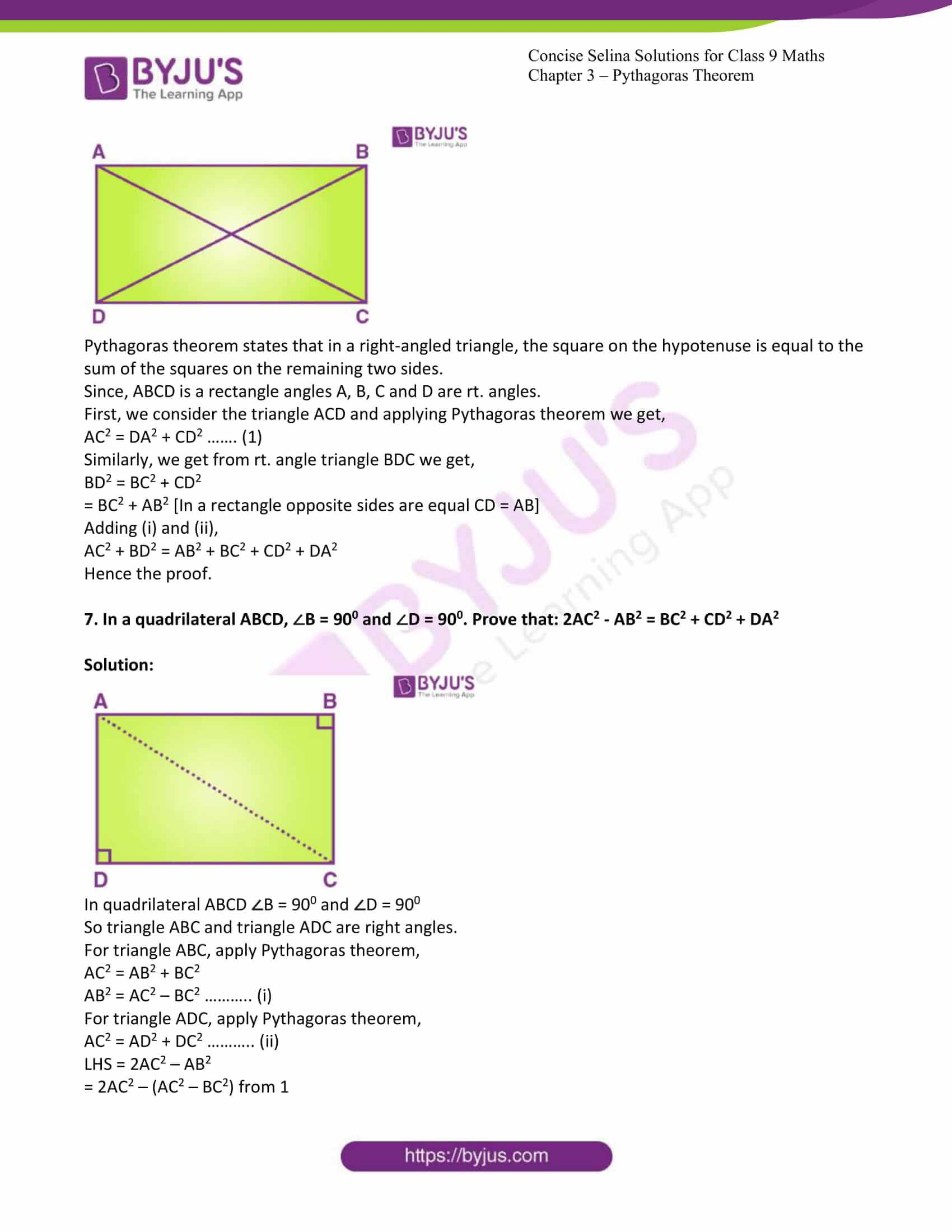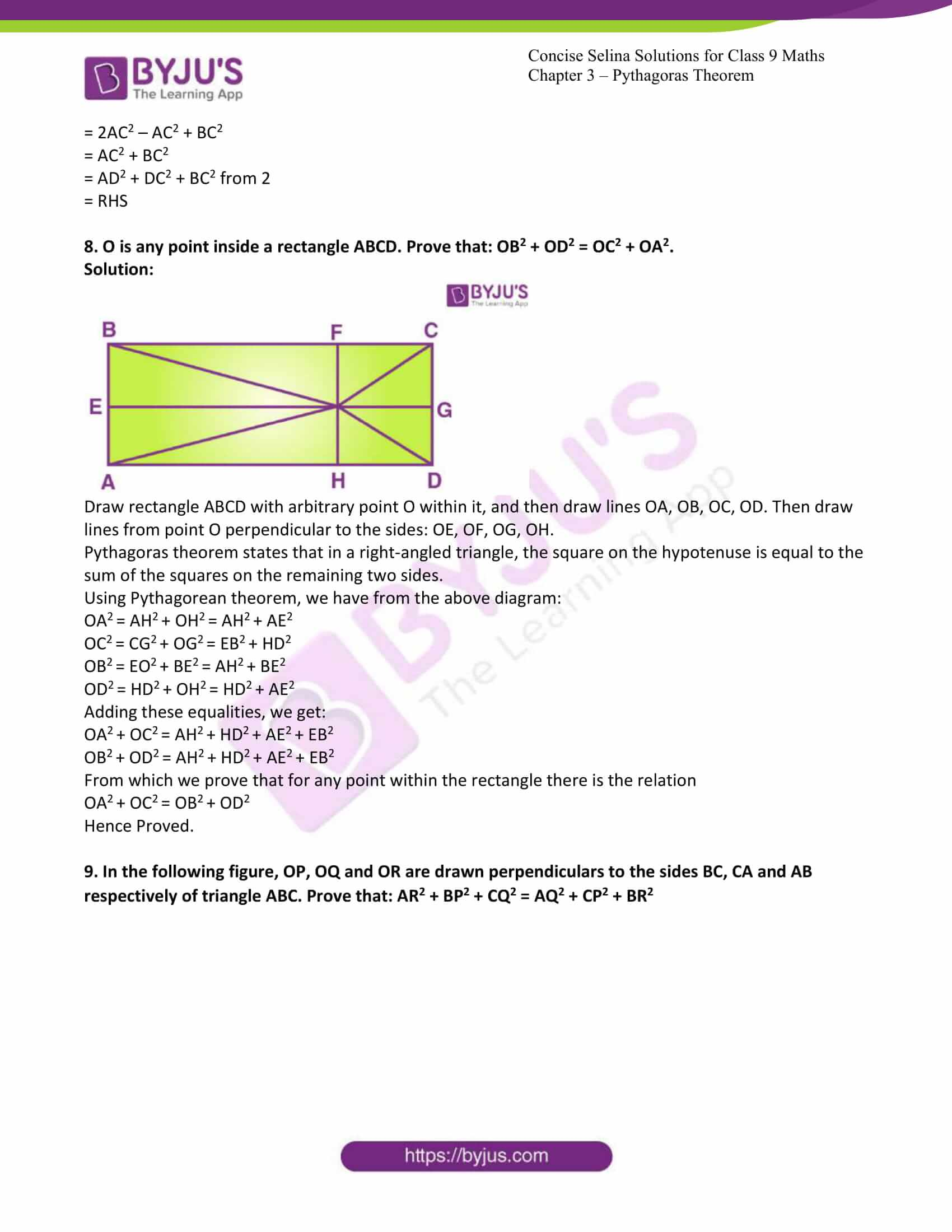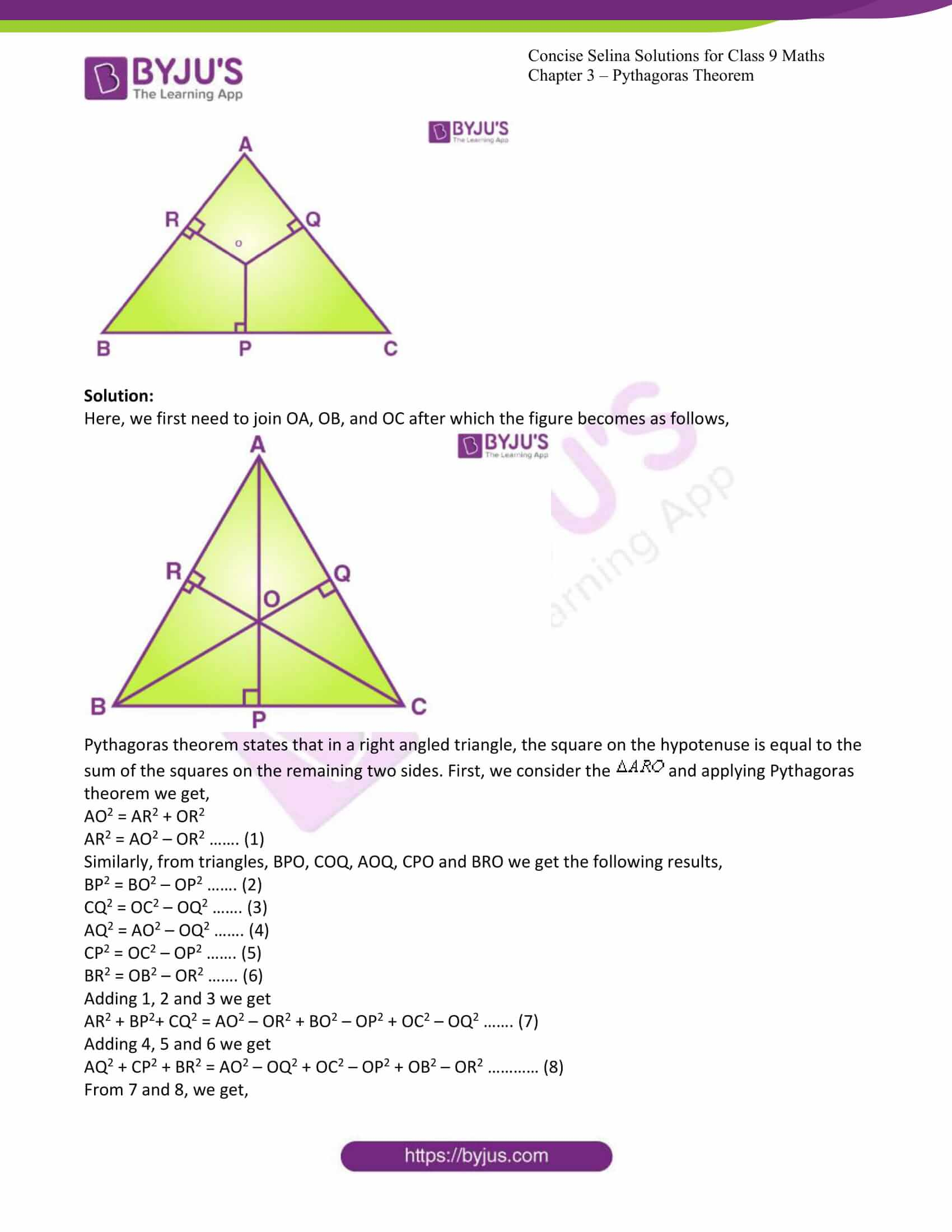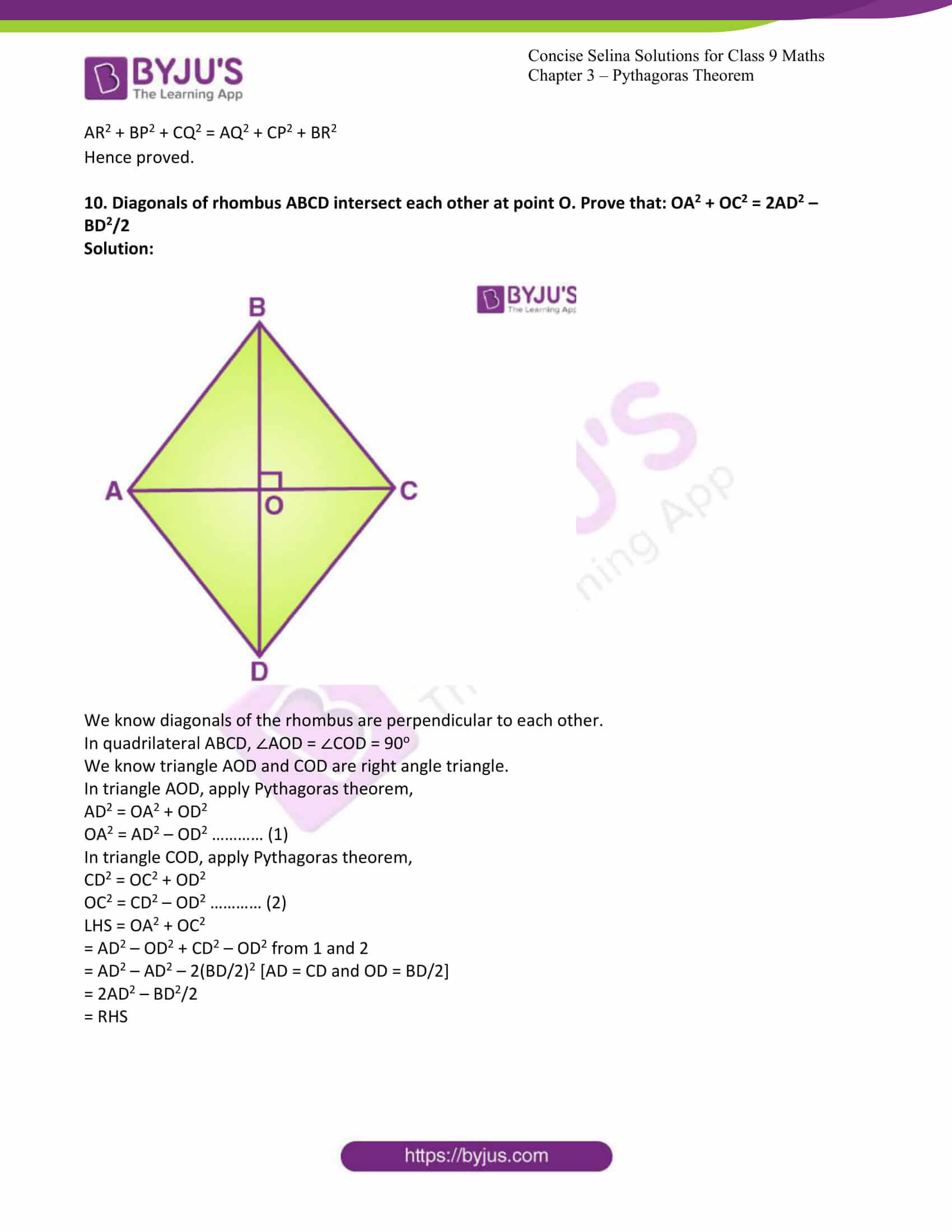Exercise 13A PAGE: 158

1. A ladder 13 m long rests against a vertical wall. If the foot of the ladder is 5 m from the foot of the wall, find the distance of the other end of the ladder from the ground.

Solution:

The pictorial representation of the given problem is given below,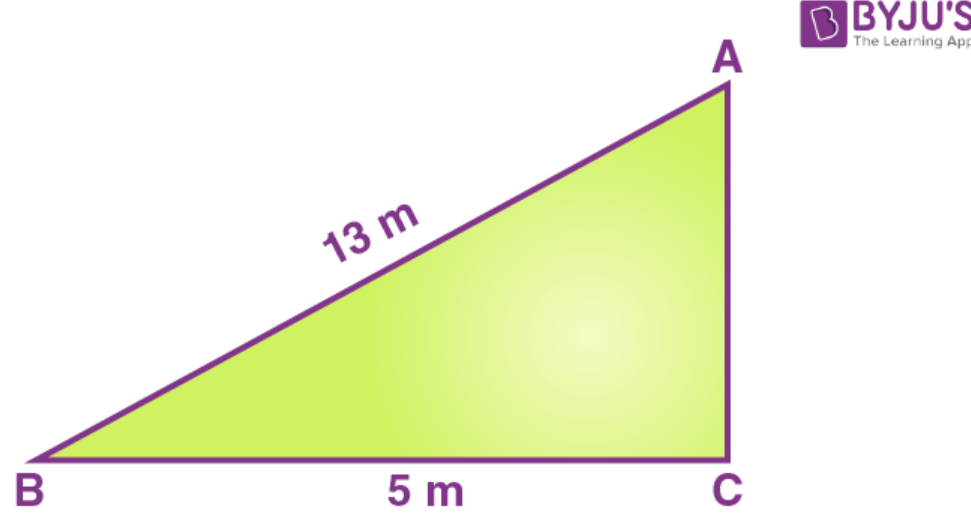Pythagoras theorem states that in a right-angled triangle, the square on the hypotenuse is equal to the sum of the squares on the remaining two sides.

(i)Here, AB is the hypotenuse. Therefore, applying the Pythagoras theorem, we get,

AB2 = BC2 + CA2

132 = 52 + CA2

CA2 = 132 – 52

CA2 = 144

CA = 12m

Therefore, the distance of the other end of the ladder from the ground is 12m

2. A man goes 40 m due north and then 50 m due west. Find his distance from the starting point.

Solution:

Here, we need to measure the distance AB as shown in the figure below,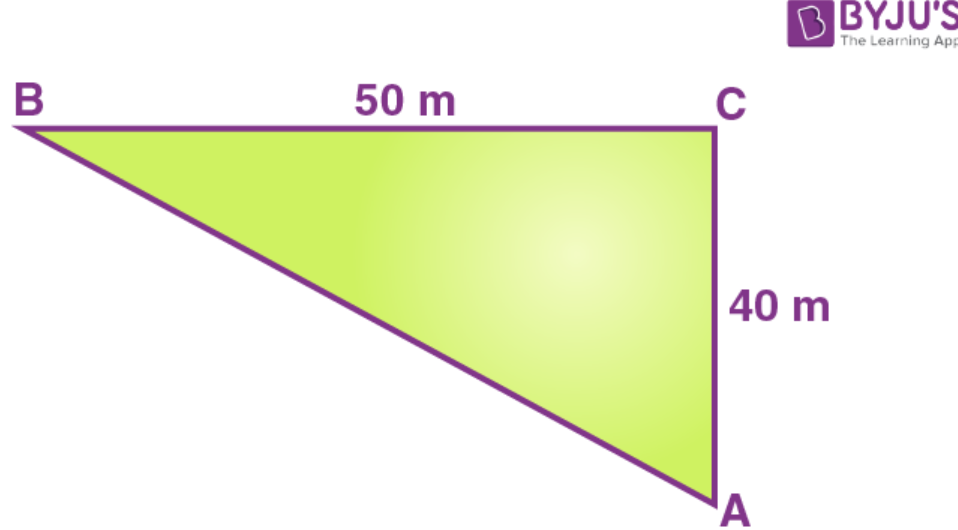Pythagoras theorem states that in a right-angled triangle, the square on the hypotenuse is equal to the sum of the squares on the remaining two sides.

Therefore, in this case

AB2 = BC2 +CA2

AB2 = 502 + 402

AB2 = 2500 + 1600

AB2 = 4100

AB = 64.03

Therefore, the required distance is 64.03 m.

3. In the figure: ∠PSQ = 90o, PQ = 10 cm, QS = 6 cm and RQ = 9 cm. Calculate the length of PR.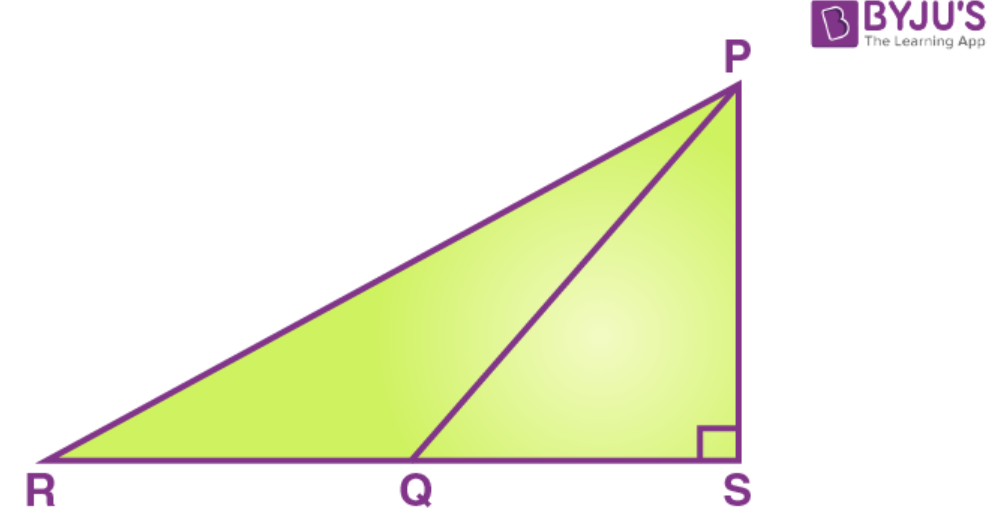Solution:

Pythagoras theorem states that in a right-angled triangle, the square on the hypotenuse is equal to the sum of the squares on the remaining two sides.

First, we consider the triangle PQS and applying Pythagoras theorem we get,

PQ2 = PS2 + QS2

102 = PS2 + 62

PS2 = 100 – 36

PS2 = 64

PS = 8

Now, we consider the triangle PRS and applying Pythagoras theorem we get,

PR2 = RS2 + PS2

PR2 = 152 + 82

PR = 17

Therefore, the length of PR = 17cm

4. The given figure shows a quadrilateral ABCD in which AD = 13 cm, DC = 12 cm, BC = 3 cm and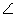ABD =BCD = 90o. Calculate the length of AB.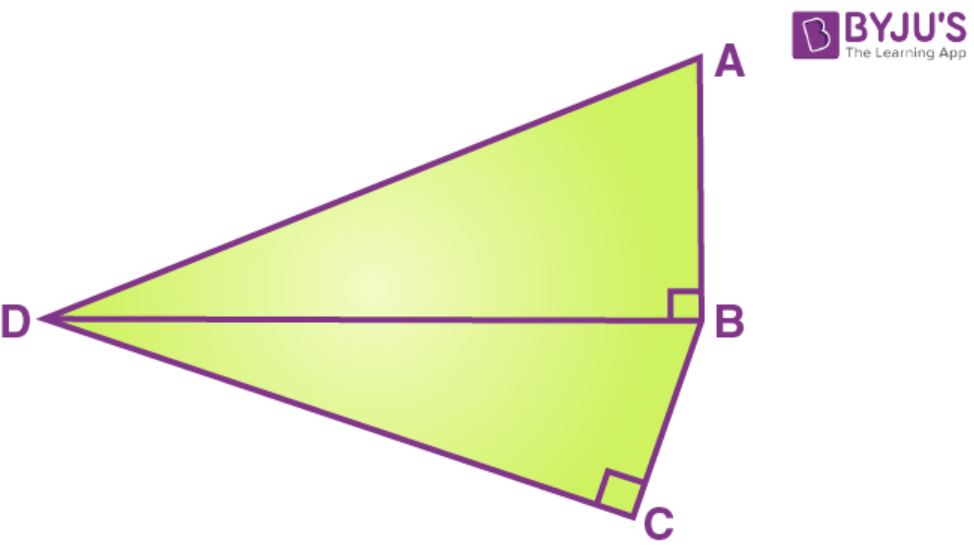Solution:

Pythagoras theorem states that in a right-angled triangle, the square on the hypotenuse is equal to the sum of the squares on the remaining two sides.

First, we consider the triangle BDC and applying Pythagoras theorem we get,

DB2 = DC2 + BC2

DB2 = 122 + 32

= 144 + 9

= 153

Now, we consider the triangle ABD and applying Pythagoras theorem we get,

DA2 = DB2 + BA2

132 = 153 + BA2

BA2 = 169 – 153

BA = 4

The length of AB is 4 cm.

5. AD is drawn perpendicular to base BC of an equilateral triangle ABC. Given BC = 10 cm, find the length of AD, correct to 1 place of decimal.

Solution:

Since ABC is an equilateral triangle therefore, all the sides of the triangle are of same measure and the perpendicular AD will divide BC in two equal parts.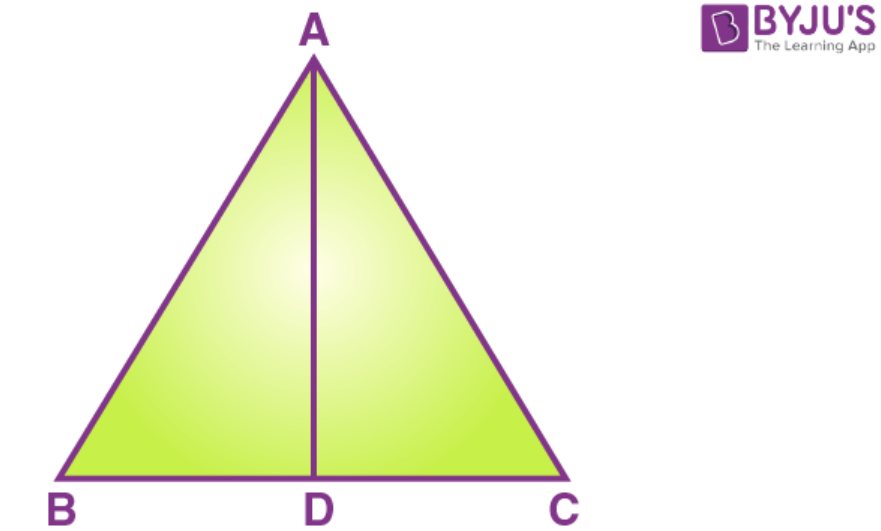Pythagoras theorem states that in a right-angled triangle, the square on the hypotenuse is equal to the sum of the squares on the remaining two sides.

Here, we consider the triangle ABD and applying Pythagoras theorem we get,

= 8.7

Therefore, the length of AD is 8.7 cm

6. In triangle ABC, given below, AB = 8 cm, BC = 6 cm and AC= 3 cm. Calculate the length of OC.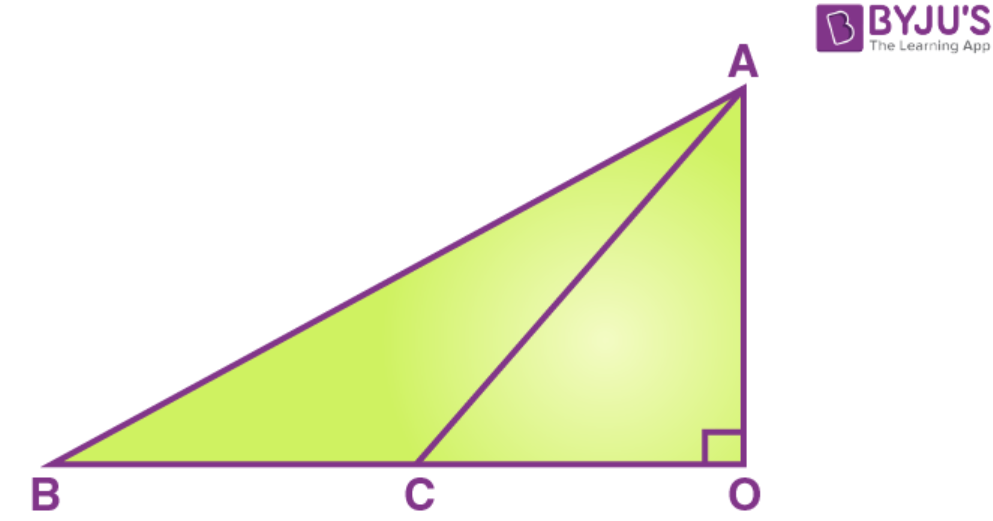Solution:

We have Pythagoras theorem which states that in a right-angled triangle, the square on the hypotenuse is equal to the sum of the squares on the remaining two sides.

First, we consider the triangle ABO and applying Pythagoras theorem we get,

AB2 = AO2 + OB2

AO2 = AB2 – OB2

AO2 = AB2 – (BC + OC)2

Let OC = x

AO2 = AB2 – (BC + x)2 ……… (1)

First, we consider the triangle ACO and applying Pythagoras theorem we get

AC2 = AO2 + x2

AO2 = AC2 – x2 ……… (2)

From 1 and 2

AB2 – (BC + x)2 = AC2 – x2

82 – (6 + x)2 = 32 – x2

X = 1 7/12 cm

Therefore, the length of OC will be 19/12 cm

7. In triangle ABC, AB = AC = x, BC = 10 cm and the area of the triangle is 60 cm2. Find x.

Solution:

Here, the diagram will be,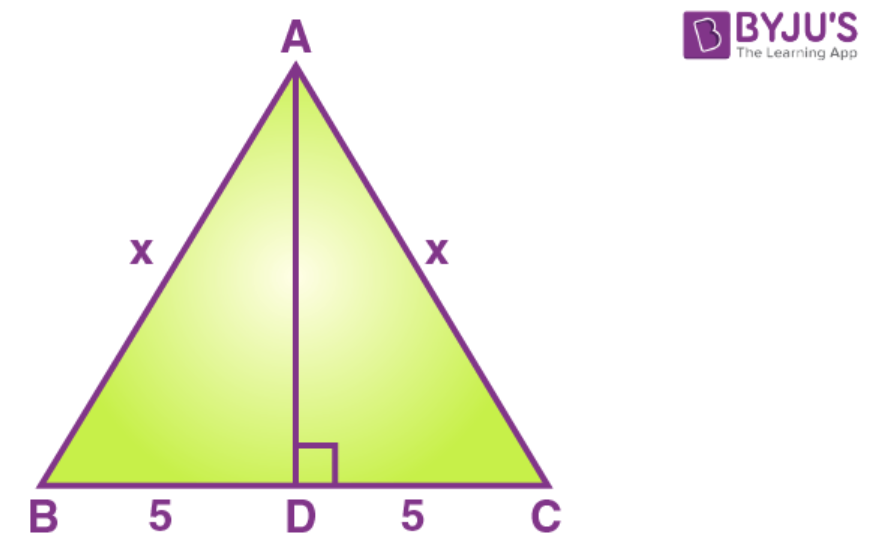We have Pythagoras theorem which states that in a right-angled triangle, the square on the hypotenuse is equal to the sum of the squares on the remaining two sides.

Since, ABC is an isosceles triangle, therefore perpendicular from vertex will cut the base in two equal segments.

First, we consider the triangle ABD and applying Pythagoras theorem we get,

AD = √(x2 – 25) ………. (1)

Now,

Area = 60

½ (10) [√(x2 – 25)] = 60

x = 13

Therefore x = 13 cm

8. If the sides of triangle are in the ratio 1 :2: 1, show that is a right-angled triangle.

Solution:

Let, the sides of the triangle be, x2 + x2 = 2x2 = (√2x)2 ……… (1)

Here, in (i) it is shown that, square of one side of the given triangle is equal to the addition of square of other two sides. This is nothing but Pythagoras theorem which states that in a right-angled triangle, the square on the hypotenuse is equal to the sum of the squares on the remaining two sides.

Therefore, the given triangle is right angled triangle.

9. Two poles of heights 6 m and 11 m stand vertically on a plane ground. If the distance between their feet is 12 m; find the distance between their tips.

Solution:

The diagram of the given problem is given below,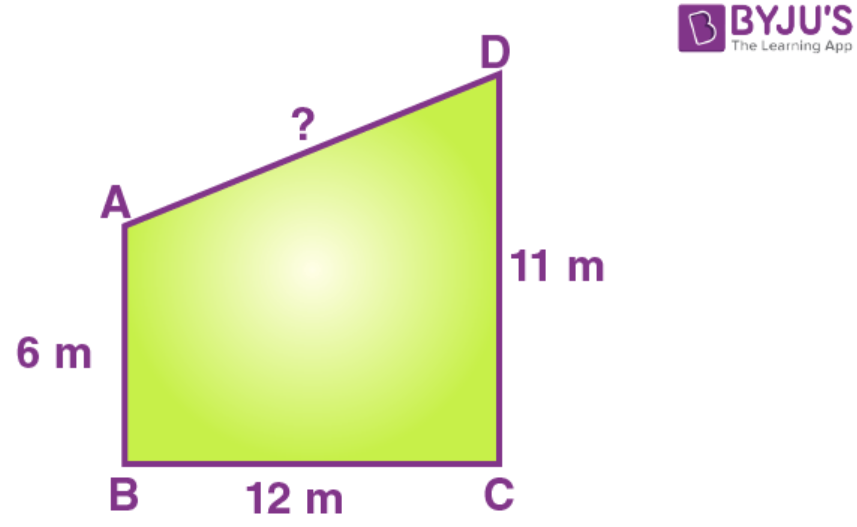We have Pythagoras theorem which states that in a right-angled triangle, the square on the hypotenuse is equal to the sum of the squares on the remaining two sides.

Here 11 – 6 = 5m

Base = 12 m

Applying Pythagoras theorem, we get

h2 = 52 + 122

= 25 + 144

= 169

h = 13

therefore, the distance between the tips will be 13m

10. In the given figure, AB//CD, AB = 7 cm, BD = 25 cm and CD = 17 cm; find the length of side BC.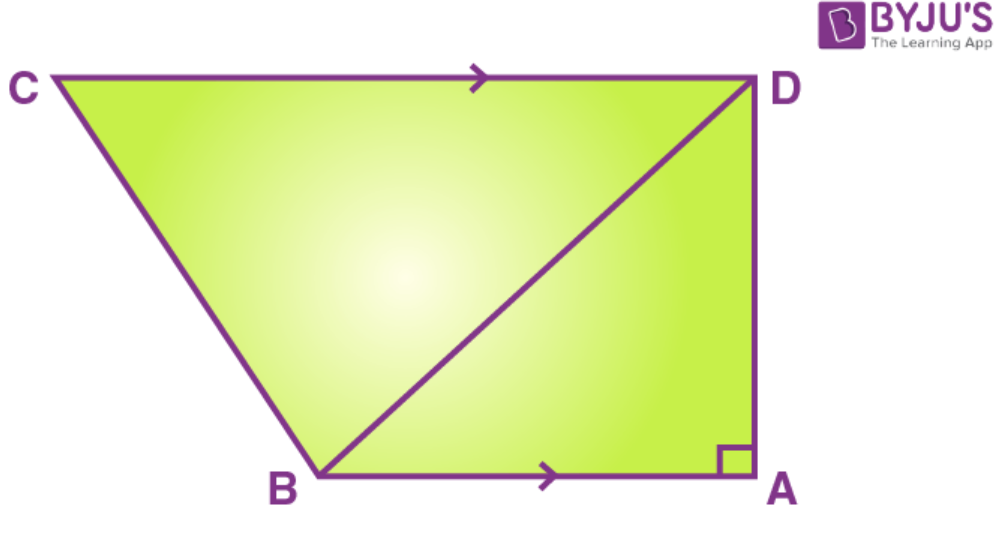Take M be the point on CD such that AB = DM.

So, DM = 7cm and MC = 10 cm

Join points B and M to form the line segment BM.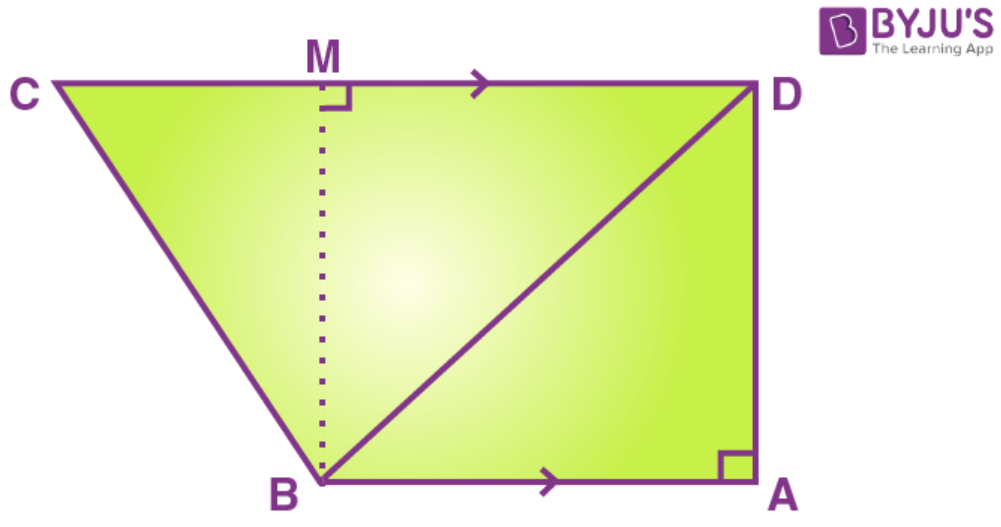In triangle CMB

CB2 = CM2 + MB2

CB2 = 102 + 242

CB2 = 676

CB = 26 cm

Exercise 13B PAGE: 163

1. In the figure, given below, AD parallel to BC. Prove that: c2 = a2 + b2 – 2ax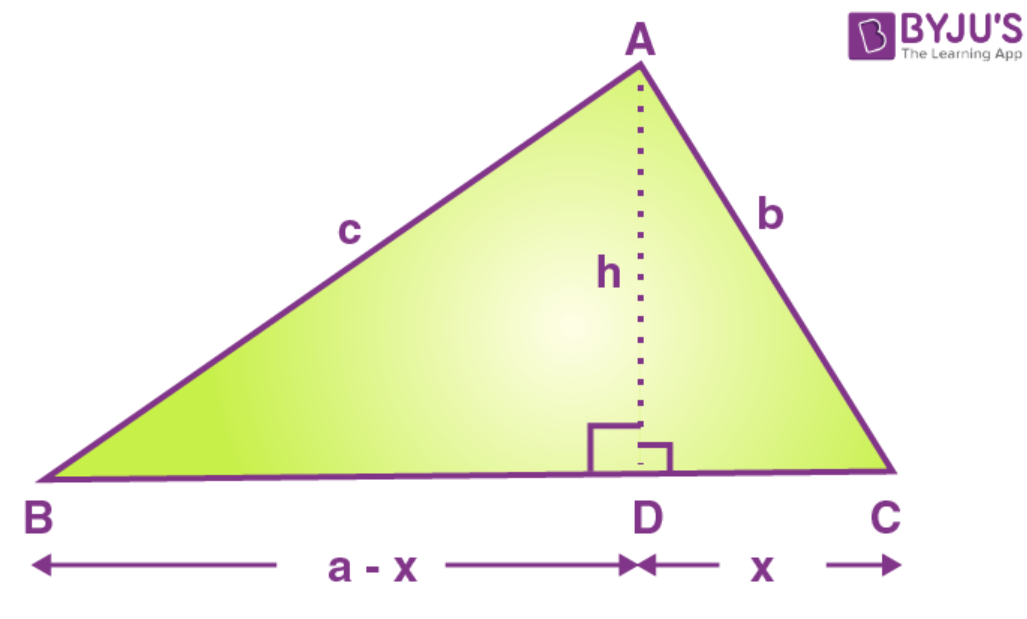Solution:

Pythagoras theorem states that in a right-angled triangle, the square on the hypotenuse is equal to the sum of the squares on the remaining two sides.

First, we consider the triangle ABD and applying Pythagoras theorem we get,

c2 = h2 + (a – x)2

h2 = c2 – (a – x)2 ……… (1)

First, we consider the triangle ACD and applying Pythagoras theorem we get

b2 = h2 + x2

h2 = b2 – x2 ………. (2)

from 1 and 2

c2 – (a – x)2 = b2 – x2

c2 – a2 – x2 + 2ax = b2 – 2ax

c2 = a2 + b2 – 2ax

hence the proof.

2. In equilateral Δ ABC, AD parallel to BC and BC = x cm. Find, in terms of x, the length of AD.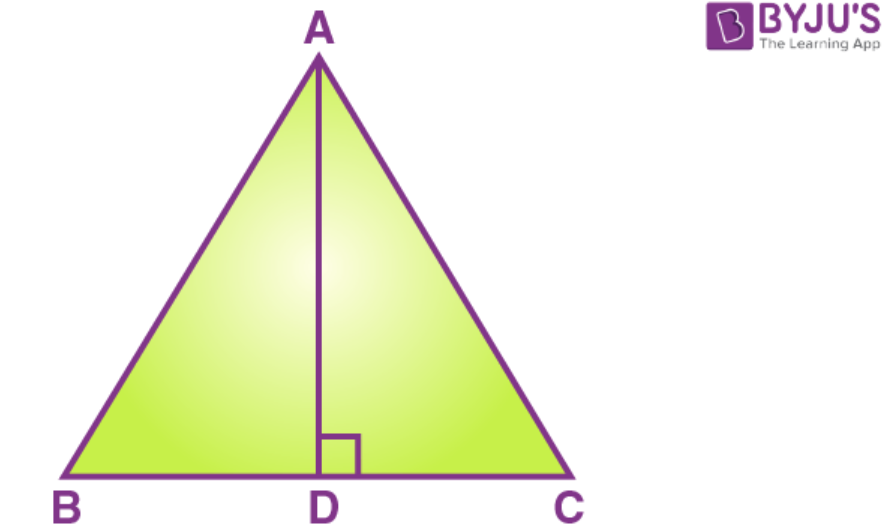Solution:

In equilateral Δ ABC, AD parallel to BC.

Therefore, BD = DC = x/2 cm.

Applying Pythagoras theorem, we get

3. ABC is a triangle, right-angled at B. M is a point on BC. Prove that:

AM2 + BC2 = AC2 + BM2.

Solution:

The pictorial form of the given problem is as follows,

Pythagoras theorem states that in a right-angled triangle, the square on the hypotenuse is equal to the sum of the squares on the remaining two sides.

First, we consider the triangle ABM and applying Pythagoras theorem we get,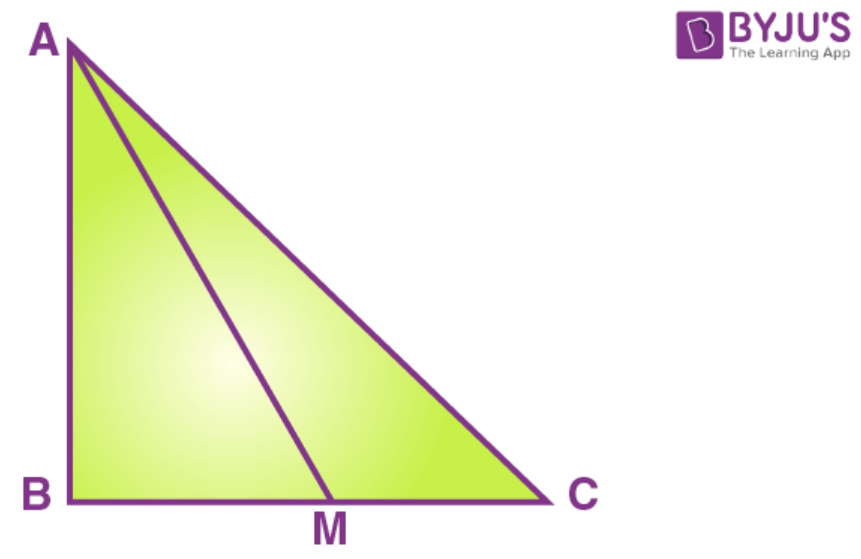AM2 = AB2 + BM2

AB2 = AM2 – BM2 ……….. (1)

Now we consider the triangle ABC and applying Pythagoras theorem we get

AC2 = AB2 + BC2

AB2 = AC2 – BC2 …… (2)

From 1 and 2 we get

AM2 – BM2 = AC2 + BM2

AM2 + BC2 = AC2 + BM2

Hence the proof.

4. M and N are the mid-points of the sides QR and PQ respectively of a triangle PQR, right-angled at Q. Prove that:

(i) PM2 + RN2 = 5 MN2

(ii) 4 PM2 = 4 PQ2 + QR2

(iii) 4 RN2 = PQ2 + 4 QR2

(iv) 4 (PM2 + RN2) = 5 PR2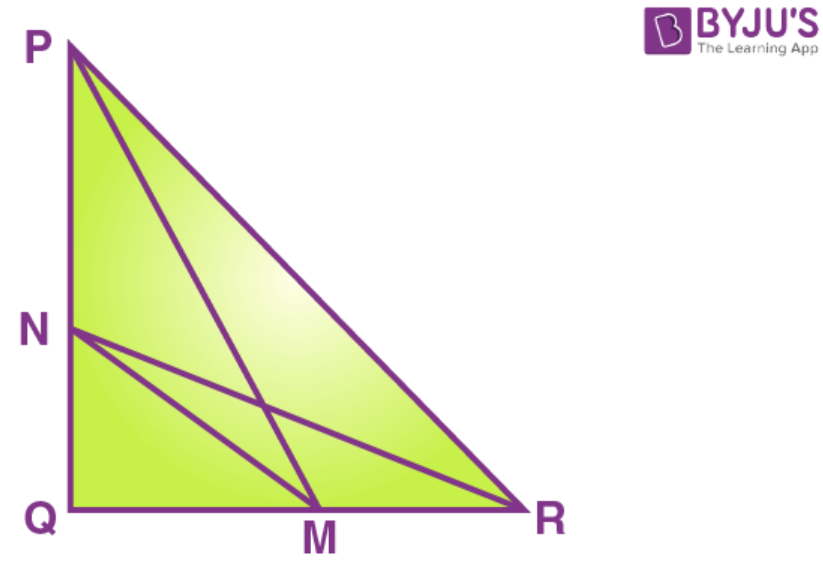Solution:

Draw, PM, MN, NR

Pythagoras theorem states that in a right-angled triangle, the square on the hypotenuse is equal to the sum of the squares on the remaining two sides.

Since, M and N are the mid-points of the sides QR and PQ respectively, therefore, PN = NQ, QM = RM

(i)

First, we consider the triangle PQM and applying Pythagoras theorem we get,

PM2 = PQ2 + MQ2

= (PN + NQ)2 + MQ2

= PN2 + NQ2 + 2 PN. NQ + MQ2

= MN2 + PN2 + 2 PN. NQ [ we know MN2 = NQ2 + MQ2] ………….. (1)

Now we consider the triangle RNQ and applying Pythagoras theorem,

RN2 = NQ2 + RQ2

= NQ2 + (QM + RM)2

= NQ2 + QM2 + 2 QM. RM + RM2 …………. (2)

Adding 1 and 2 we get

PM2 + RN2 = MN2 + PN2 + 2PN. NQ + MN2 + RM2+ 2QM. RM

PM2 + RN2 = 2MN2 + PN2 + RM2 + 2PN. NQ + 2QM. RM

PM2 + RN2 = 2MN2 + NQ2 + QM2 + 2(QN)2 + 2 (QM)2

PM2 + RN2 = 2MN2 + MN2 + 2MN2

PM2 + RN2 = 5MN2

Hence the proof.

(ii) Now consider the triangle PQM and apply Pythagoras theorem we get

PM2 = PQ2 + MQ2

4PM2 = 4PQ2 + 4 MQ2 [multiplying both sides by 4]

4PM2 = 4PQ2 + 4 (½ QR2) [MQ = ½ QR]

4PM2 = 4PQ2 + QR2

Hence the proof.

(iii) now consider triangle RQN and apply Pythagoras theorem we get

RN2 = NQ2 + RQ2

4RN2 = 4NQ2 + 4 QR2 [multiplying both sides by 4]

4RN2 = 4QR2 + 4 (½ PQ2) [NQ = ½ PQ]

4RN2 = PQ2 + 4QR2

Hence the proof.

(iv) now consider the triangle PQM and apply Pythagoras theorem,

PM2 = PQ2 + MQ2

= (PN + NQ)2 + MQ2

= PN2 + NQ2 + 2 PN. NQ + MQ2

= MN2 + PN2 + 2 PN. NQ [ we know MN2 = NQ2 + MQ2] ………….. (1)

Now we consider the triangle RNQ and applying Pythagoras theorem,

RN2 = NQ2 + RQ2

= NQ2 + (QM + RM)2

= NQ2 + QM2 + 2 QM. RM + RM2

= MN2 + RM2 + 2 QM. RM …………. (2)

Adding 1 and 2 we get

PM2 + RN2 = MN2 + PN2 + 2PN. NQ + MN2 + RM2+ 2QM. RM

PM2 + RN2 = 2MN2 + PN2 + RM2 + 2PN. NQ + 2QM. RM

PM2 + RN2 = 2MN2 + NQ2 + QM2 + 2(QN)2 + 2 (QM)2

PM2 + RN2 = 2MN2 + MN2 + 2MN2

PM2 + RN2 = 5MN2

4 (PM2+ RN2) = 4. 5 (NQ2 + MQ2]

4 (PM2+ RN2) = 4. 5 [(½ PQ)2 + (½ QR)2]

4 (PM2+ RN2) = 5PR2

Hence the proof.

5. In triangle ABC, ∠B = 90o and D is the mid-point of BC. Prove that: AC2 = AD2 + 3CD2.Solution:

Pythagoras theorem states that in a right-angled triangle, the square on the hypotenuse is equal to the sum of the squares on the remaining two sides.

In triangle ABC, B = 90o and D is the mid-point of BC. Join AD. Therefore, BD=DC

First, we consider the triangle ADB and applying Pythagoras theorem we get,

AB2 = AD2 – BD2 …. (1)

Similarly, we get from rt. angle triangles ABC we get,

AC2 = AB2 + BC2

AB2 = AC2 – BC2 …. (1)

From 1 and 2 we get

AC2 – BC2 = AD2 – BD2

AC2 = AD2 – BD2 + BC2

AC2 = AD2 – CD2 + 4CD2 [BD = CD = ½ BC]

Hence the proof.

6. In a rectangle ABCD, prove that: AC2 + BD2 = AB2 + BC2 + CD2 + DA2.

Solution: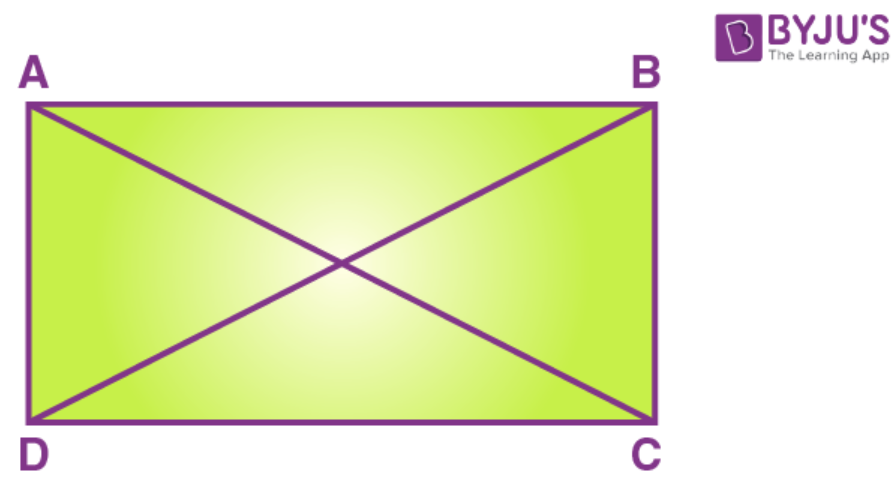Pythagoras theorem states that in a right-angled triangle, the square on the hypotenuse is equal to the sum of the squares on the remaining two sides.

Since, ABCD is a rectangle angles A, B, C and D are rt. angles.

First, we consider the triangle ACD and applying Pythagoras theorem we get,

AC2 = DA2 + CD2 ……. (1)

Similarly, we get from rt. angle triangle BDC we get,

BD2 = BC2 + CD2

= BC2 + AB2 [In a rectangle opposite sides are equal CD = AB]

AC2 + BD2 = AB2 + BC2 + CD2 + DA2

Hence the proof.

7. In a quadrilateral ABCD, ∠B = 900 and ∠D = 900. Prove that: 2AC2 – AB2 = BC2 + CD2 + DA2

Solution: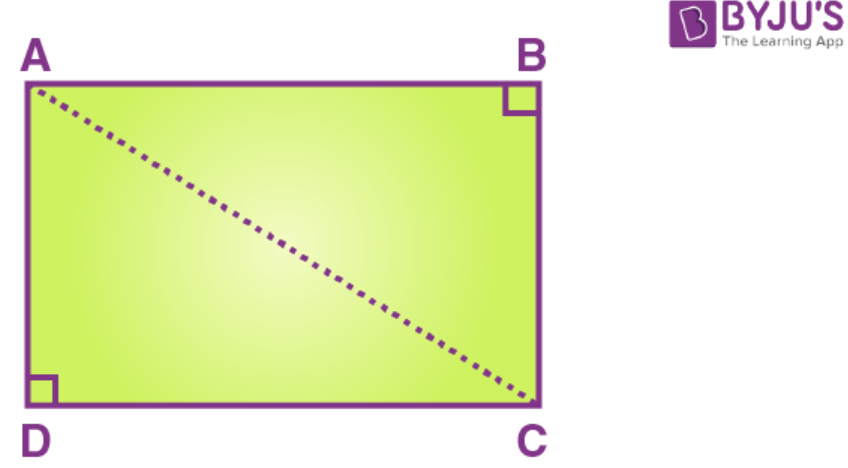In quadrilateral ABCD B = 900 and D = 900

So triangle ABC and triangle ADC are right angles.

For triangle ABC, apply Pythagoras theorem,

AC2 = AB2 + BC2

AB2 = AC2 – BC2 ……….. (i)

For triangle ADC, apply Pythagoras theorem,

AC2 = AD2 + DC2 ……….. (ii)

LHS = 2AC2 – AB2

= 2AC2 – (AC2 – BC2) from 1

= 2AC2 – AC2 + BC2

= AC2 + BC2

= AD2 + DC2 + BC2 from 2

= RHS

8. O is any point inside a rectangle ABCD. Prove that: OB2 + OD2 = OC2 + OA2.

Solution: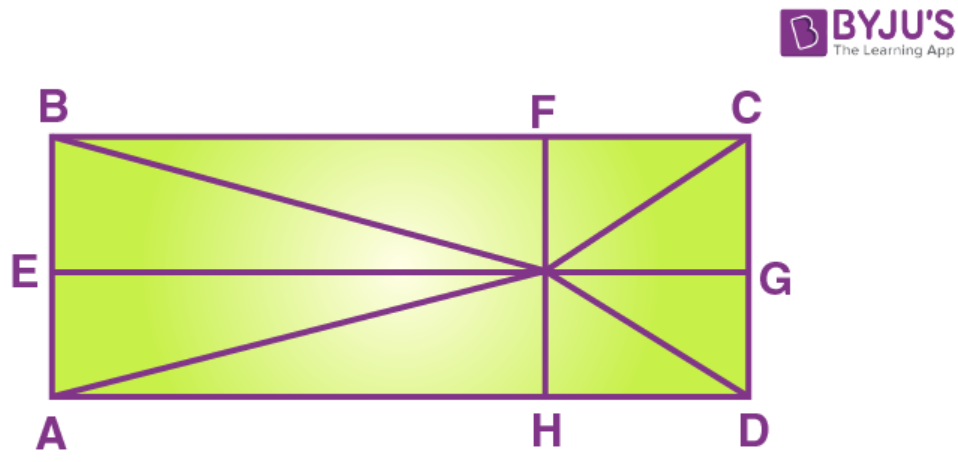Draw rectangle ABCD with arbitrary point O within it, and then draw lines OA, OB, OC, OD. Then draw lines from point O perpendicular to the sides: OE, OF, OG, OH.

Pythagoras theorem states that in a right-angled triangle, the square on the hypotenuse is equal to the sum of the squares on the remaining two sides.

Using Pythagorean theorem, we have from the above diagram:

OA= AH+ OH= AH+ AE2

OC= CG+ OG= EB+ HD2

OB= EO+ BE= AH+ BE2

OD= HD+ OH= HD+ AE2

OA+ OC= AH+ HD+ AE+ EB2

OB+ OD= AH+ HD+ AE+ EB2

From which we prove that for any point within the rectangle there is the relation

OA+ OC= OB+ OD2

Hence Proved.

9. In the following figure, OP, OQ and OR are drawn perpendiculars to the sides BC, CA and AB respectively of triangle ABC. Prove that: AR2 + BP2 + CQ2 = AQ2 + CP2 + BR2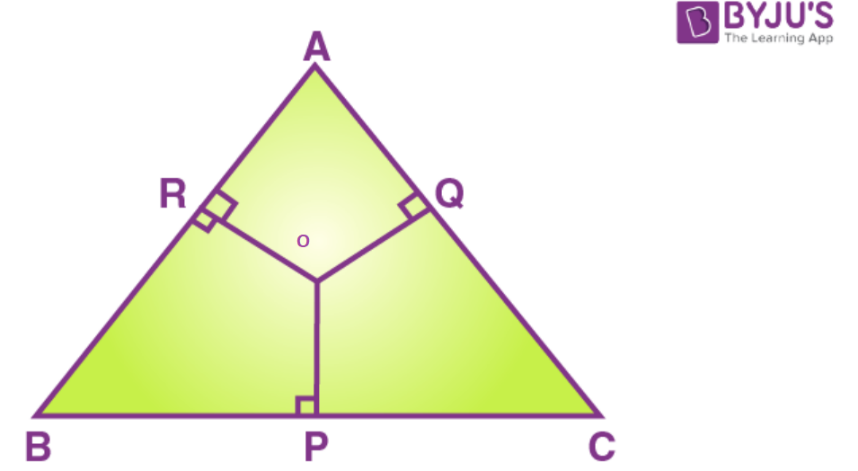Solution:

Here, we first need to join OA, OB, and OC after which the figure becomes as follows,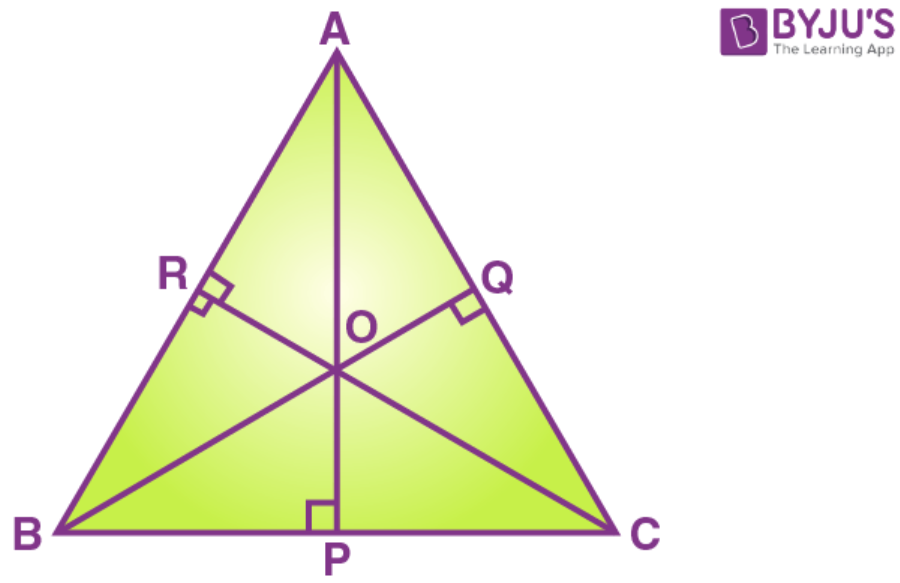Pythagoras theorem states that in a right angled triangle, the square on the hypotenuse is equal to the sum of the squares on the remaining two sides. First, we consider the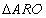and applying Pythagoras theorem we get,

AO2 = AR2 + OR2

AR2 = AO2 – OR2 ……. (1)

Similarly, from triangles, BPO, COQ, AOQ, CPO and BRO we get the following results,

BP2 = BO2 – OP2 ……. (2)

CQ2 = OC2 – OQ2 ……. (3)

AQ2 = AO2 – OQ2 ……. (4)

CP2 = OC2 – OP2 ……. (5)

BR2 = OB2 – OR2 ……. (6)

Adding 1, 2 and 3 we get

AR2 + BP2+ CQ2 = AO2 – OR2 + BO2 – OP2 + OC2 – OQ2 ……. (7)

Adding 4, 5 and 6 we get

AQ2 + CP2 + BR2 = AO2 – OQ2 + OC2 – OP2 + OB2 – OR2 ………… (8)

From 7 and 8, we get,

AR2 + BP2 + CQ2 = AQ2 + CP2 + BR2

Hence proved.

10. Diagonals of rhombus ABCD intersect each other at point O. Prove that: OA2 + OC2 = 2AD2 – BD2/2

Solution: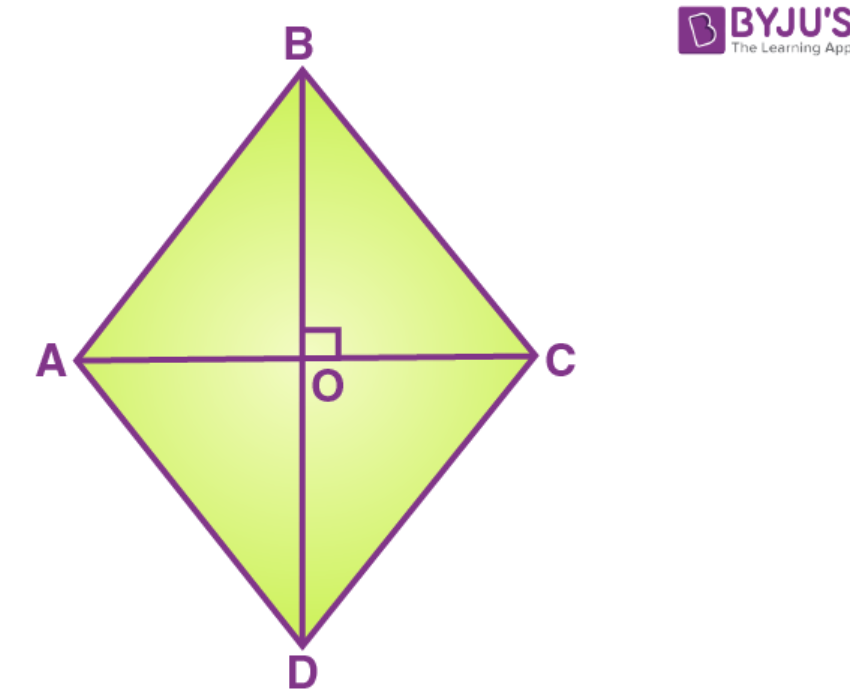We know diagonals of the rhombus are perpendicular to each other.

In quadrilateral ABCD, ∠AOD = ∠COD = 90o

We know triangle AOD and COD are right angle triangle.

In triangle AOD, apply Pythagoras theorem,

OA2 = AD2 – OD2 ………… (1)

In triangle COD, apply Pythagoras theorem,

CD2 = OC2 + OD2

OC2 = CD2 – OD2 ………… (2)

LHS = OA2 + OC2

= AD2 – OD2 + CD2 – OD2 from 1 and 2

= RHS

## Selina Solutions for Class 9 Maths Chapter 13- Pythagoras Theorem

The Chapter 13, Pythagoras Theorem is composed of 2 exercises and the solutions given here contain answers to all the questions present in these exercises. Let us have a look at some of the topics that are being discussed in this chapter.

13.1 Introduction

13.2 Pythagoras Theorem

1. Area based: In a right-angled triangle, the square on the hypotenuse is equal to the sum of the squares on the remaining two sides.
2. Alternate: In a right-angled triangle, the square of the hypotenuse is equal to the sum of the squares on the remaining two sides.

## Selina Solutions for Class 9 Maths Chapter 13- Pythagoras Theorem

In Chapter 13 of Class 9, the students are taught about the Pythagoras Theorem. This chapter also belongs to the unit Triangle. The chapter helps students in understanding the proof and simple applications of the pythagoras theorem as well as its converse. Study the Chapter 13 of Selina textbook to understand more about Pythagoras Theorem. Learn the Selina Solutions for Class 9 effectively to come out with flying colours in the examinations.